# You are instructed to create 400. mL of a 0.39 M phosphate buffer with a pH...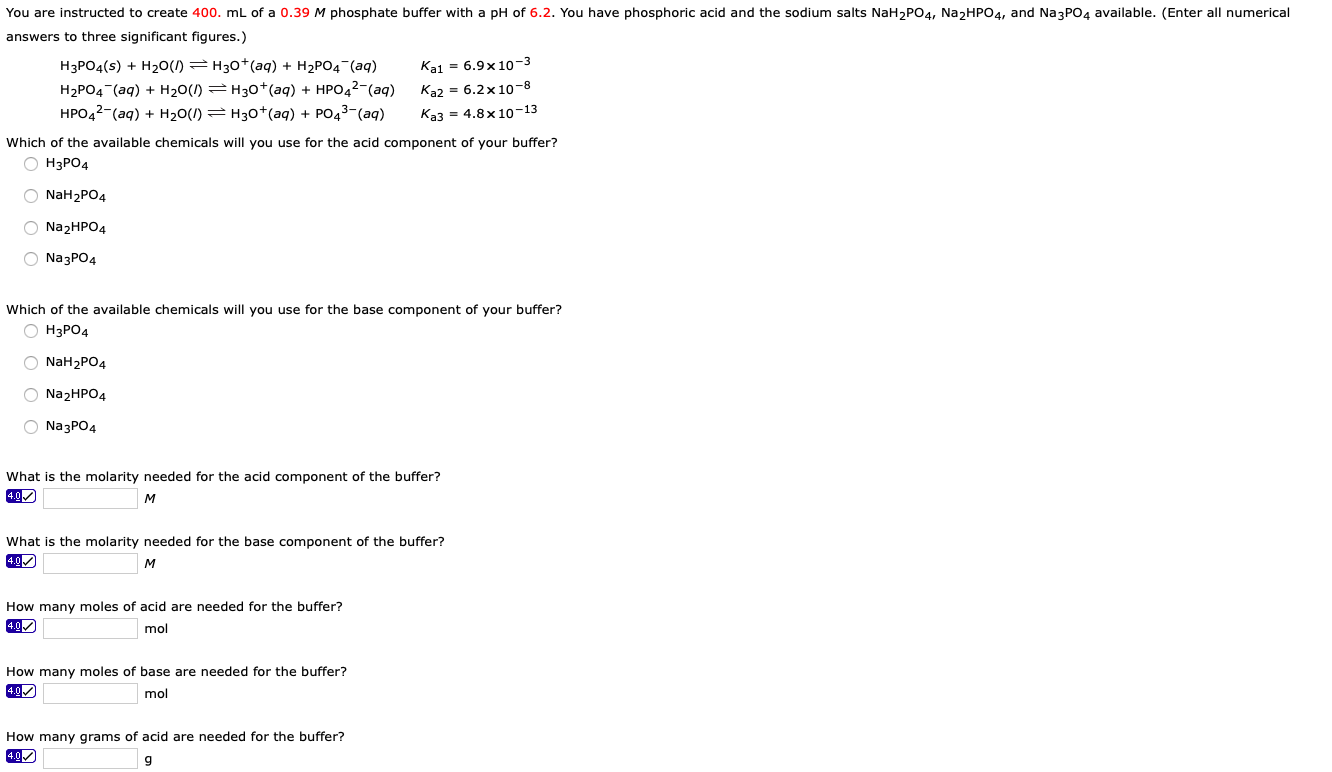You are instructed to create 400. mL of a 0.39 M phosphate buffer with a pH of 6.2. You have phosphoric acid and the sodium salts NaH2PO4, Na2HPO4, and Na3PO4 available. (Enter all numerical answers to three significant figures.) H3PO4(s) + H20(I) =H30+ (aq) + H2PO4 (aq) Kai = 6.9x10-3 H2PO4 (aq) + H20(1) =H30+ (aq) + HPO42-(aq) Ka2 = 6.2 x 10-8 HPO42-(aq) + H20(1) = H30+(aq) + PO43-(aq) Ka3 = 4.8x10-13 Which of the available chemicals will you use for the acid component of your buffer? ОН3РО4 NaH2PO4 Na2HPO4 Na3PO4 Which of the available chemicals will you use for the base component of your buffer? OH3PO4 NaH2PO4 Na2HPO4 Na3PO4 What is the molarity needed for the acid component of the buffer? What is the molarity needed for the base component of the buffer? How many moles of acid are needed for the buffer? mol How many moles of base are needed for the buffer? mol How many grams of acid are needed for the buffer?

Question 1. NaH2PO4

Explanation: pH is close to pKa2

Question 2. Na2HPO4

Explanation: pH is close to pKa2

Note: pKa2 = -Log(Ka2) = -Log(6.2*10-8) = 7.2

#### Earn Coin

Coins can be redeemed for fabulous gifts.

Similar Homework Help Questions
• ### You are instructed to create 400. mL of a 0.39 M phosphate buffer with a pH...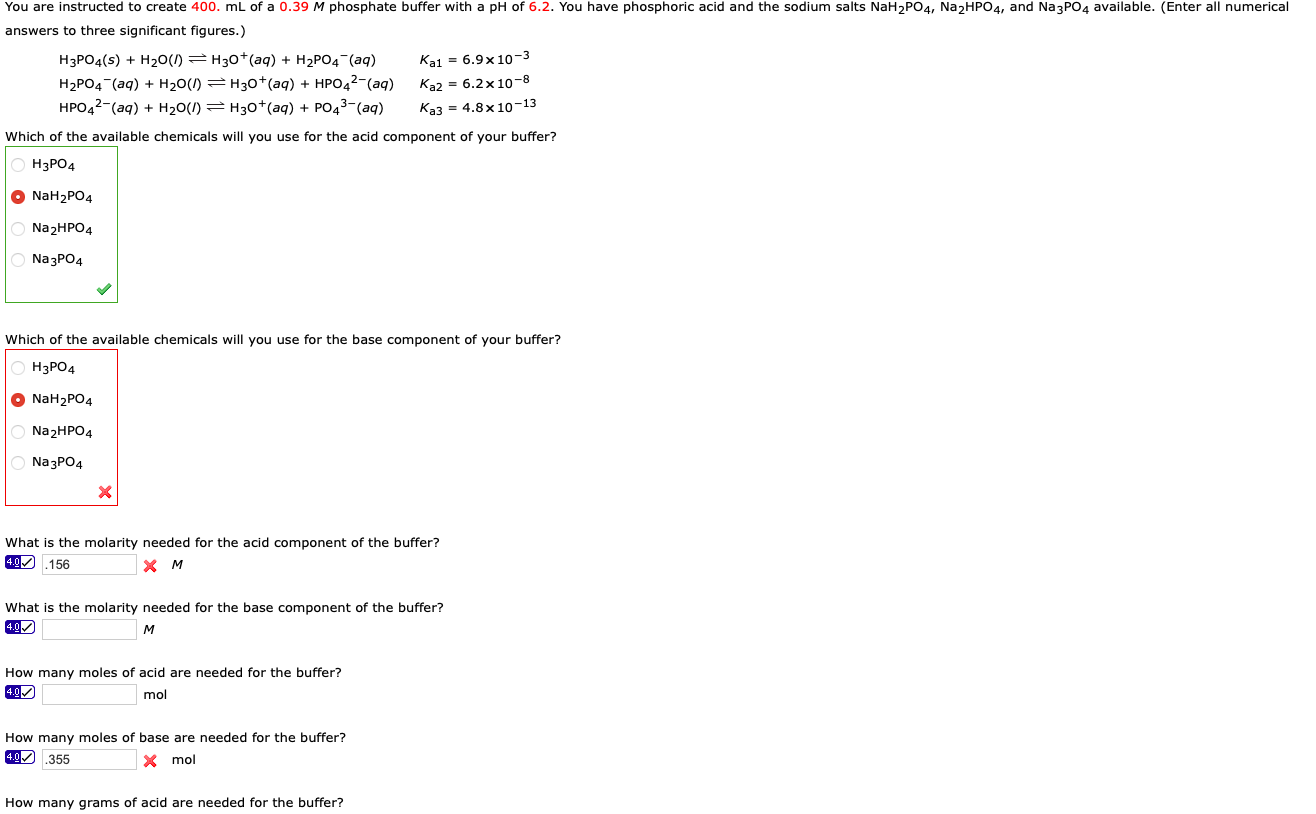You are instructed to create 400. mL of a 0.39 M phosphate buffer with a pH of 6.2. You have phosphoric acid and the sodium salts NaH2PO4, Na2HPO4, and Na3PO4 available. (Enter all numerical answers to three significant figures.) H3PO4(s) + H20(1) =H30+ (aq) + H2PO4 (aq) Kai = 6.9x10-3 H2PO4 (aq) + H20() = H30+(aq) + HPO42- (aq) Ka2 = 6.2x 10-8 HPO42-(aq) + H20(I) = H30+(aq) + PO43-(aq) Ka3 = 4.8 x 10-13 Which of the available chemicals...

• ### You are instructed to create 400. mL of a 0.39 M phosphate buffer with a pH...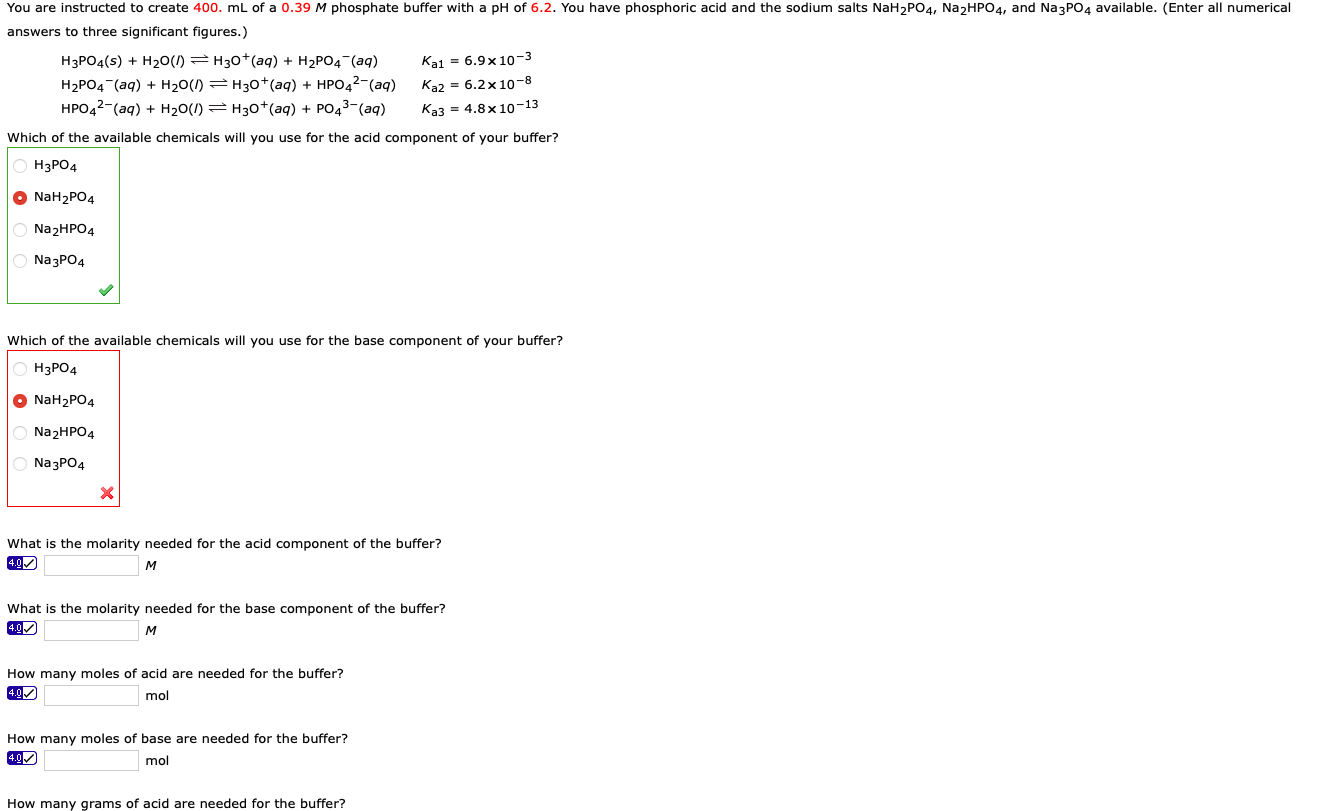You are instructed to create 400. mL of a 0.39 M phosphate buffer with a pH of 6.2. You have phosphoric acid and the sodium salts NaH2PO4, Na2HPO4, and Na3PO4 available. (Enter all numerical answers to three significant figures.) H3PO4(s) + H2O(l) = H30+ (aq) + H2P04 (aq) Kai = 6.9 x 10-3 H2PO4 (aq) + H20(1) =H30+ (aq) + HPO42-(aq) Ka2 = 6.2 x 10-8 HPO42-(aq) + H20(I) = H30+(aq) + PO43-(aq) Ka3 = 4.8 x 10-13 Which of...

• ### You are instructed to create 400. mL of a 0.40 M phosphate buffer with a pH...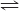You are instructed to create 400. mL of a 0.40 M phosphate buffer with a pH of 6.4. You have phosphoric acid and the sodium salts NaH2PO4, Na2HPO4, and Na3PO4 available. (Enter all numerical answers to three significant figures.) H3PO4(s) + H2O(l) H3O+(aq) + H2PO4−(aq)     Ka1 = 6.9 ✕ 10−3 H2PO4−(aq) + H2O(l) H3O+(aq) + HPO42−(aq)     Ka2 = 6.2 ✕ 10−8 HPO42−(aq) + H2O(l) H3O+(aq) + PO43−(aq) Ka3 = 4.8 ✕ 10−13 Which of the available chemicals will you use...

• ### You are instructed to create 200. mL of a 0.63 M phosphate buffer with a pH...

You are instructed to create 200. mL of a 0.63 M phosphate buffer with a pH of 6.0. You have phosphoric acid and the sodium salts NaH2PO4, Na2HPO4, and Na3PO4 available. (Enter all numerical answers to three significant figures.) H3PO4(s) + H2O(l) equilibrium reaction arrow H3O+(aq) + H2PO4−(aq) Ka1 = 6.9 ✕ 10−3 H2PO4−(aq) + H2O(l) equilibrium reaction arrow H3O+(aq) + HPO42−(aq) Ka2 = 6.2 ✕ 10−8 HPO42−(aq) + H2O(l) equilibrium reaction arrow H3O+(aq) + PO43−(aq) Ka3 = 4.8 ✕ ...

• ### You are instrucbed to create 900. mL of a 0.59 M phosphate buffer with a pH...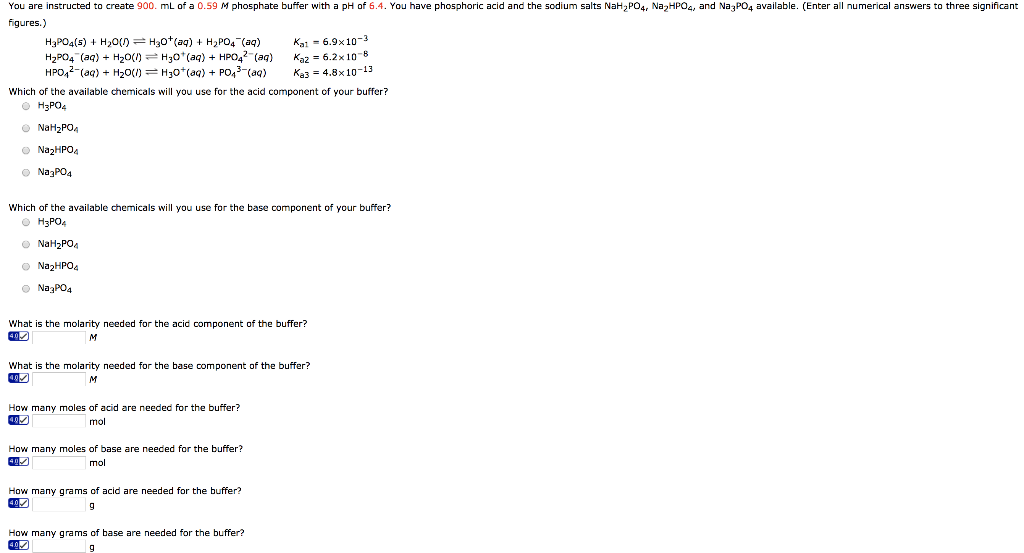You are instrucbed to create 900. mL of a 0.59 M phosphate buffer with a pH of 6.4. You have phosphoric acid and the sodium salts NaH2PO4, Na2HPO4, and Na3PO4 available. numerical answers to three significant H3PO4(s)H20)H3 aH2PO4(aq) H2PO4-(aq) + H2O(りーH3O + (aq) + HP042-(aq) Ka2-6.2×10-8 HPO()H20()H3)PO43 (aq) Ka 4.8x10-13 Ka 6.9x10-2 Which of the available chemicals will you use for the acid component of your buffer? O H3PO4 O Na2HPO Na3PO4 Which of the available chemicals will you use...

• ### You are instructed to create 180. mL of a 0.06 M Acetate buffer with a pH of 4.0. You have Acetic acid and the sodium sa...

You are instructed to create 180. mL of a 0.06 M Acetate buffer with a pH of 4.0. You have Acetic acid and the sodium salt NaC2H3O2, available. (Enter all numerical answers to three significant figures.) HC2H3O2(s) + H2O(l) equilibrium reaction H3O+(aq) + C2H3O2−(aq)     Ka1 = 1.7 ✕ 10−5 What is the molarity needed for the base component of the buffer? How many moles of base are needed for the buffer? How many grams of base are needed for the...

• ### Buffer Practice 1.) You wish to prepare 750 mL of a buffer solution of pH =...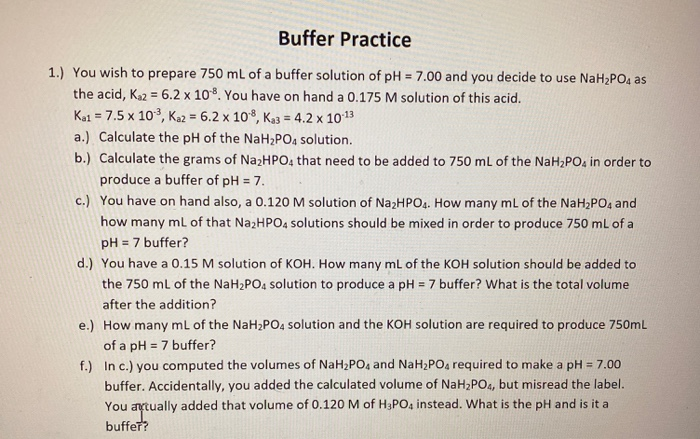Buffer Practice 1.) You wish to prepare 750 mL of a buffer solution of pH = 7.00 and you decide to use NaH2PO4 as the acid, K2 = 6.2 x 10. You have on hand a 0.175 M solution of this acid. Kai = 7.5 x 103, Ka2 = 6.2 x 10-8, K3 = 4.2 x 10-13 a.) Calculate the pH of the NaH2PO4 solution. b.) Calculate the grams of Na2HPO4 that need to be added to 750 mL of...

• ### Design a buffer that has a pH of 4.77 using one of the weak acid/conjugate base...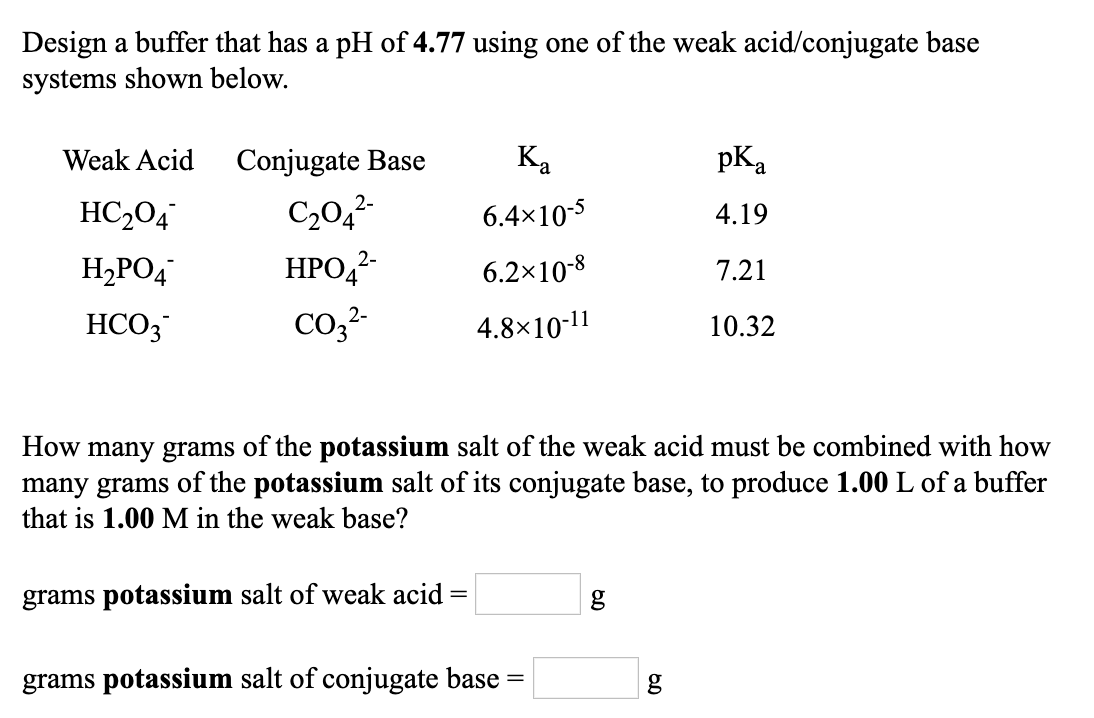Design a buffer that has a pH of 4.77 using one of the weak acid/conjugate base systems shown below. Weak Acid Conjugate Base Ka pKa HC2O4- C2O42- 6.4×10-5 4.19 H2PO4- HPO42- 6.2×10-8 7.21 HCO3- CO32- 4.8×10-11 10.32 How many grams of the potassium salt of the weak acid must be combined with how many grams of the potassium salt of its conjugate base, to produce 1.00 L of a buffer that is 1.00 M in the weak base? grams potassium...

• ### Given: pH: 7.60 Concentration (M): 0.050 mL: 100mL Determine the Mass of Each Component Recall that...

Given: pH: 7.60 Concentration (M): 0.050 mL: 100mL Determine the Mass of Each Component Recall that buffers are formed from conjugate acid/base pairs. Using the information given about your assigned buffer, determine how much of each component (acid and base) you will need in order to prepare it in the lab. (This will require a system of equations because there are two “unknowns.”) Note: the conjugate acid in this case is H2PO4−, and the conjugate base is HPO42−. 1. Using...

• ### Given: pH: 7.60 Concentration (M): 0.050 mL: 100mL Determine the Mass of Each Component Recall that...

Given: pH: 7.60 Concentration (M): 0.050 mL: 100mL Determine the Mass of Each Component Recall that buffers are formed from conjugate acid/base pairs. Using the information given about your assigned buffer, determine how much of each component (acid and base) you will need in order to prepare it in the lab. (This will require a system of equations because there are two “unknowns.”) Note: the conjugate acid, in this case, is H2PO4−, and the conjugate base is HPO42−. Equations 1...